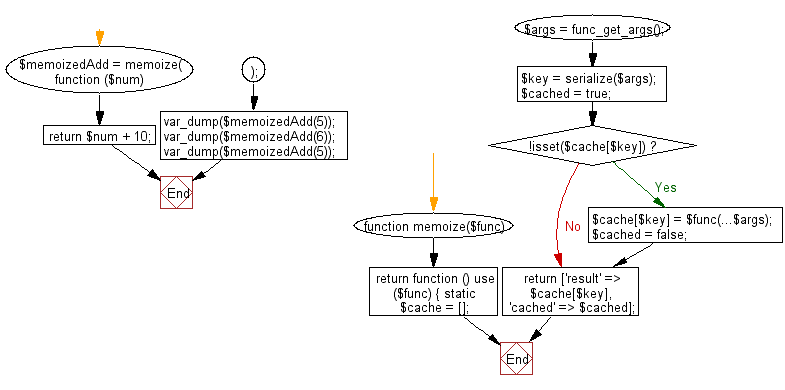﻿ PHP Exercise: Memoize a given function results in memory - w3resource

# PHP Exercises: Memoize a given function results in memory

## PHP: Exercise-99 with Solution

Write a PHP program to memoize a given function results in memory.

Note: In computing, memoization or memoisation is an optimization technique used primarily to speed up computer programs by storing the results of expensive function calls and returning the cached result when the same inputs occur again.

Sample Solution: -

PHP Code:

``````<?php
//Licence: https://bit.ly/2CFA5XY
function memoize(\$func)
{
return function () use (\$func) {
static \$cache = [];

\$args = func_get_args();
\$key = serialize(\$args);
\$cached = true;

if (!isset(\$cache[\$key])) {
\$cache[\$key] = \$func(...\$args);
\$cached = false;
}

return ['result' => \$cache[\$key], 'cached' => \$cached];
};
}

function (\$num) {
return \$num + 10;
}
);

?>
```
```

Sample Output:

```array(2) {
["result"]=>
int(15)
["cached"]=>
bool(false)
}
array(2) {
["result"]=>
int(16)
["cached"]=>
bool(false)
}
array(2) {
["result"]=>
int(15)
["cached"]=>
bool(true)
}
```

Flowchart:PHP Code Editor:

Have another way to solve this solution? Contribute your code (and comments) through Disqus.

What is the difficulty level of this exercise?

Test your Programming skills with w3resource's quiz.

﻿

## PHP: Tips of the Day

Mutates the original array to filter out the values specified

Example:

```<?php
function tips_pull(&\$items, ...\$params)
{
\$items = array_values(array_diff(\$items, \$params));
return \$items;
}

\$items = ['x', 'y', 'z', 'x', 'y', 'z'];
print_r(tips_pull(\$items, 'y', 'z'));
?>
```

Output:

```Array
(
 => x
 => x
)
```